Courses

# Test: Zeroes And Coefficients

## 20 Questions MCQ Test Mathematics (Maths) Class 10 | Test: Zeroes And Coefficients

Description
This mock test of Test: Zeroes And Coefficients for Class 10 helps you for every Class 10 entrance exam. This contains 20 Multiple Choice Questions for Class 10 Test: Zeroes And Coefficients (mcq) to study with solutions a complete question bank. The solved questions answers in this Test: Zeroes And Coefficients quiz give you a good mix of easy questions and tough questions. Class 10 students definitely take this Test: Zeroes And Coefficients exercise for a better result in the exam. You can find other Test: Zeroes And Coefficients extra questions, long questions & short questions for Class 10 on EduRev as well by searching above.
QUESTION: 1

### If α and β are the zeroes of the polynomial 5x2 – 7x + 2, then sum of their reciprocals is:

Solution:

We have 2 find (1/α + 1/β)

now 1/α + 1/β = (α + β)/ α β (taking LCM)

now by the given poly. we get

(α + β) = -b/a = 7/5

α β = c/a = 2/5

so, (α + β)/ α β = (7/5) / (2/5)

= 7/2

So, 1/α + 1/β = (α + β)/ α β = 7/2

Hence, 1/α + 1/β = 7/2

QUESTION: 2

### If one zero of polynomial f(x) = (k2 + 4)x2 + 13x + 4k is reciprocal of the other, then k =

Solution: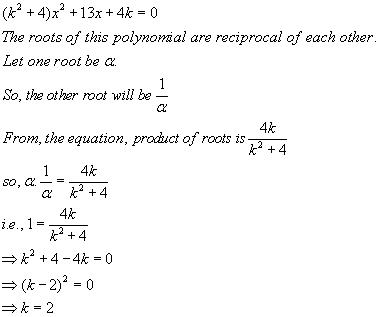QUESTION: 3

### Find the quadratic polynomial whose zeros are 2 and -6

Solution:

We know that quadratic equation is of the form x2-(sum of zeros)x+product of zeros
Sum of zeros=2-6=-4
Product of zeros=2*(-6)=-12
So the equation is x2+4x-12

QUESTION: 4

If α, β, γ be the zeros of the polynomial p(x) such that α + β + γ = 3, αβ + βγ + γα = 10 and αβγ = -24, then p(x) is

Solution:
QUESTION: 5

If -√5 and √5 are the roots of the quadratic polynomial. Find the quadratic polynomial.

Solution:

We have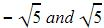as the roots which means x +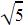and x-are the factors of the quadratic equation. Multiplying x+and x-and applying a2-bwe get the equation x2-5.

QUESTION: 6

If one root of polynomial equation ax2 + bx + c = 0 be reciprocal of other, then

Solution: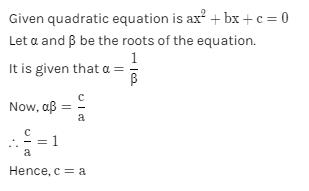QUESTION: 7

If one zero of the polynomial x2 + kx + 18 is double the other zero then k = ?

Solution:

Let one zero of the equation be x
Then, other would be 2x
As we know,
x × 2x = 18
x2 = 9
x = 3, -3
Case 1:
If x = 3, 2x = 6
Therefore, -k = sum of roots = 9
⇒ k = -9
Case 2:
If x = -3, 2x = -6
Therefore, -k = -9
⇒ k = 9
So, k can be 9,-9. Therefore, option 'b' is correct.

QUESTION: 8

If one zero of 2x2 – 3x + k is reciprocal to the other, then the value of k is :​

Solution:

Given: 2x²-3x+k .............eq 1

Let the 2 zeroes be α & 1/α

Quadratic form : ax²+bx+c.........eq 2

On comparing eq 1 & eq 2 we get

a=2 ,b = -3, c= k

Product of zeroes = α × 1/α = 1

Product of zeroes= c/a

1= k/ 2

K= 2
The value of k is 2.

QUESTION: 9

Sum and the product of zeroes of the polynomial x2 +7x +10 is

Solution:

x2 + 7x + 10 = (x + 2)(x + 5)

So, the value of x2 + 7x + 10 is zero when x + 2 = 0 or x + 5 = 0

Therefore, the zeroes of x2 + 7x + 10 are –2 and –5.

Sum of zeroes = -7 = –(Coefficient of x) / (Coefficient of x2)

Product of zeroes = 10 = Constant term / Coefficient of x2

QUESTION: 10

If sum of the zeroes of the polynomial is 4 and their product is 4, then the quadratic polynomial is

Solution:

Sum of zeros= α+β =4
Product of zeros=αβ=4
We have Quadratic equation as
x2-(Sum of zeros)x+Product of zeros
=x2-4x+4

QUESTION: 11

What value/s can x take in the expression k(x – 10) (x + 10) = 0 where k is any real number.

Solution:

k(x – 10) (x + 10) =0
⇒ either k=0
Or x-10=0
Or x+10=0
Since we don’t know the value of k
So either x-10=0
x=10
Or x+10=0
x=-10
So values of x can be 10,-10

QUESTION: 12

Find the sum and the product of zeroes of the polynomial x2 +7x +10

Solution:

x2 + 7x + 10 = (x + 2)(x + 5)

So, the value of x2 + 7x + 10 is zero when x + 2 = 0 or x + 5 = 0

Therefore, the zeroes of x2 + 7x + 10 are –2 and –5.

Sum of zeroes = -7 = –(Coefficient of x) / (Coefficient of x2)

Product of zeroes = 10 = Constant term / Coefficient of x2

QUESTION: 13

The sum and product of zeros of a quadratic polynomial are 2 and -15 respectively. The quadratic polynomial is

Solution:
QUESTION: 14

If the product of two zeros of the polynomial f(x) = 2x3 + 6x2 – 4x + 9 is 3, then its third zero is

Solution:

Step-by-step explanation:

p(x) = 2x³ + 6x² – 4x + 9

Define α,β and γ

Let α,β and γ be the 3 roots

αβ = 3 (Given)

Find the products of all the zeros:

αβγ = - d/a

αβγ = - 9/2

Find the third zeros:

Given αβ = 3

3γ = -9/2

γ = -9/2 ÷ 3

γ = -3/2

The third zero is -3/2

QUESTION: 15

If α, β are zeroes of the polynomial f(x) = x2 + 5x + 8, then value of (α + β) is

Solution:

We have quadratic equation of the form
x- (sum of zeros) x + product of zeros, so comparing this with the given equation we have sum of zeros = -5
So, α + β = -5

QUESTION: 16

If sum of the squares of zeros of the quadratic polynomial f(x) = x2 – 8x + k is 40, find the value of k.​

Solution:

p (x)= x^2-8x+k
p (x)=Ax^2+Bx+C(The equation is in this form)

Let the zeroes be 'a' and 'b'

It is given that
=> a^2+b^2=40
=> (a+b)^2 - 2ab = 40 ----1

sum of zeroes
=> a+b = -B/A = -(-8)/1 = 8

Product of zeroes
=> a*b = C/A  = k

Substitute these values in the equation1 we get
=> 8^2 - 2k = 40
=> 64 - 2k = 40
=> 2k = 24
=> k = 12

Therefore the value of k is 12

QUESTION: 17

If α , β are the zeroes of f(x) = px2 – 2x + 3p and α + β = αβ then the value of p is:​

Solution:

The given polynomial is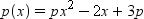Also, α and β are the zeros of p(x).

and  α + β = α × β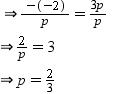QUESTION: 18

If the two zeroes of the quadratic polynomial 7x2 – 15x – k are reciprocals of each other, the value of k is:​

Solution: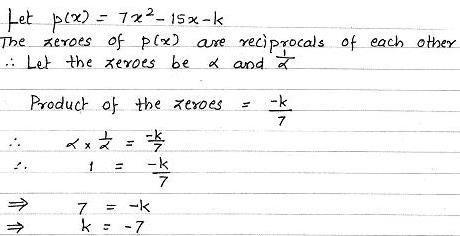QUESTION: 19

The number of polynomials having zeroes -2 and 5 is:

Solution:

Since the question doesn’t say that there are only 2 zeros of the polynomial we , there can be n number of polynomials which have two of its zeros as -2 and 5 .So the correct answer is more than 3.

QUESTION: 20

Find the sum and the product of the zeroes of the polynomial: x2-3x-10​

Solution:

X²-3x-10

x² -(5x-2x)-10

x² - 5x+2x-10

x(x-5)+2(x-5)

(x-5)(x+2)

x=5

x=-2

Sum of zeroes = α+β = 5-2 = 3
α+β = -b/a = -(-3)/1 = 3

Product of zeroes = αβ = 5*-2 = -10

αβ = c/a = -10/1 = -10

• Test